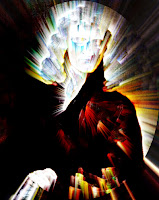## Monday, January 21, 2008

### Physics and Sir Issac NewtonInteresting that I have been taking a beginning physics course. Again, I apply an image of Sir Issac Newton produced in Photoshop using a couple of filters applied to Text.

Sir Issac Newton has been credited with the invention of Physics -- which is the science of how things work in our world. Although this is not quite true. Aristotle and other Natural Philosophers before him actually applied science to the physical world before Sir Issac Newton did so. However, the good Knight had worked hard in discovering a certain amount of things.

And in no particular order, the first was Calculus. That body of mathematics that calculated how nature worked. Newton discovered integral Calculus, the same Calculus that Archimedes used to calculate the volume of a sphere by slicing it into an infinite amount of slices.

Second was his work on optics. Third, and perhaps his most famous works, was his work on motion which is found in most every beginning Physics text.

The laws are stated as thus:

First Law
If no net force acts on a particle, then it is possible to select a set of reference frames, called inertial reference frames, observed from which the particle moves without any change in velocity. This law is often simplified into the sentence "An object will stay at rest or continue at a constant velocity unless acted upon by an external unbalanced force".
Second Law
Observed from an inertial reference frame, the net force on a particle is proportional to the time rate of change of its linear momentum: F = d[mv] / dt. Momentum is the product of mass and velocity. This law is often stated as F = ma (the net force on an object is equal to the mass of the object multiplied by its acceleration).

Third Law
Whenever a particle A exerts a force on another particle B, B simultaneously exerts a force on A with the same magnitude in the opposite direction. The strong form of the law further postulates that these two forces act along the same line. This law is often simplified into the sentence "Every action has an equal and opposite reaction".
From the investigations of these forces, it is clear that Newton had studied the "Masters" the scientists that came before him. Anaximenes, Anaxagoras, Pythagoras, and Aristotle are among those that he read. It was from their notes handed down to us by Hypatia of Alexandria that formed the seeds for the Theory of Gravitation and the Laws of Motion that Sir Issac Newton put forward.

As for my beginning physics course, Newton is lauded and praised in the History of Science. But my course material stinks. The textbook puts me to sleep, and it looks like it has been written to impress Plato and other academics of that school. Sadly, it puts them to sleep.

Nevertheless, Newton had made great strides in making the world of Physics a better place to learn and study so that we will know how our world works.